Related Articles
How to find the Cross-section of Pandas Data frame?
• Last Updated : 25 Oct, 2020

Sometimes we need to find the cross-section of pandas series or data frame. Here cross-section means getting values at the specified index, values at several indexes, values at several indexes and levels or values at the specified column and axis etc. There is a function known as pandas.DataFrame.xs() which will help in this condition.

pandas.DataFrame.xs() takes a key argument in order to select data at the particular level in MultiIndex and returns cross-section from pandas data frame.

Syntax: DataFrame.xs(key, axis=0, level=None, drop_level=True)

Parameters:
key – Label contained in the index, or partially in a MultiIndex.
axis – Axis to retrieve cross-section on.
level – In case of a key partially contained in a MultiIndex, indicate which levels are used.
drop_level – If False, returns an object with the same levels as self.

Returns:
Cross-section from the original DataFrame

Below is the pandas code which will help in the proper understanding of pandas.DataFrame.xs() function.

## Python3

 `# importing pandas library``import` `pandas as pd`` ` `# Creating a Dictionary``animal_dict ``=` `{``'num_of_legs'``: [``4``, ``0``, ``4``, ``2``, ``2``, ``2``],``                ` `               ``'num_of_wings'``: [``0``, ``0``, ``0``, ``2``, ``2``, ``2``],``                ` `               ``'class'``: [``'Reptiles'``, ``'Reptiles'``, ``'Reptiles'``,``                         ``'Birds'``, ``'Birds'``, ``'Birds'``],``                ` `               ``'animal'``: [``'Turtle'``, ``'Snake'``, ``'Crocodile'``,``                          ``'Parrot'``, ``'Owl'``, ``'Hummingbird'``],``                ` `               ``'locomotion'``: [``'swim_walk'``, ``'swim_crawl'``, ``'swim_walk'``, ``                              ``'flies'``, ``'flies'``, ``'flies'``]}`` ` `# Converting to Data frame and setting index``df ``=` `pd.DataFrame(data``=``animal_dict)``df ``=` `df.set_index([``'class'``, ``'animal'``, ``'locomotion'``])`` ` `# Displaying Data frame``df`

Output: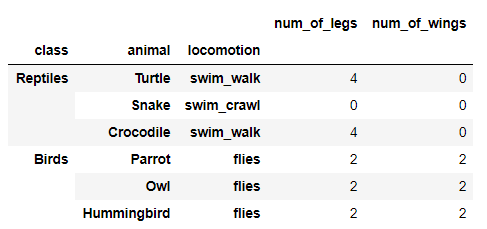Example 1: Getting values at a specific index

## Python3

 `# Using dataframe.xs() function``# to get values of a specific index ``df.xs(``'Reptiles'``)`

Output: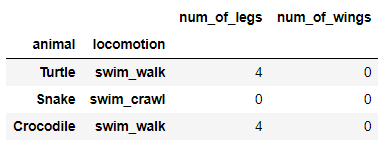Example 2: Getting values at several indexes

## Python3

 `# Using dataframe.xs() function``# to get values at several indexes ``df.xs((``'Birds'``, ``'Parrot'``))`

Output: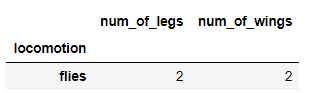Example 3: Getting values at the specified index and level

## Python3

 `# Using dataframe.xs() function``# to get values at specified index``# and level``df.xs(``'Crocodile'``, level ``=` `1``)`

Output: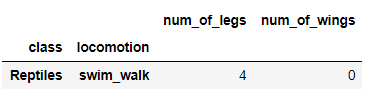Example 4: Getting values at several indexes and levels

## Python3

 `# Using dataframe.xs() function``# to get values at several indexes``# and levels``df.xs((``'Birds'``, ``'flies'``),``      ``level``=``[``0``, ``'locomotion'``])`

Output: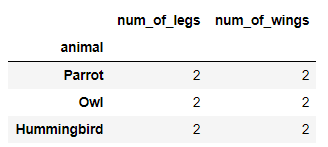Example 5: Getting values at the specified column and axis

## Python3

 `# Using dataframe.xs() function``# to get values at specified column``# and axis``df.xs(``'num_of_wings'``, axis``=``1``)`

Output: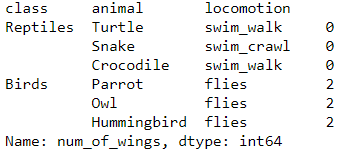Attention geek! Strengthen your foundations with the Python Programming Foundation Course and learn the basics.

To begin with, your interview preparations Enhance your Data Structures concepts with the Python DS Course. And to begin with your Machine Learning Journey, join the Machine Learning – Basic Level Course

My Personal Notes arrow_drop_up